# Numerical Study of a Longitudinal Solar Dryer in Semi-Arid Area; Case Study

Numerical Study of a Longitudinal Solar Dryer in Semi-Arid Area; Case Study

Sofiane KherrourAmor Bouhdjar Salah Hanini Abdelhamid Boualit Djemoui Lalmi

Unité de Recherche Appliquée en Energies Renouvelables, URAER, Centre de Développement des Energies Renouvelables, CDER, 47133, Ghardaïa, Algeria

Biomaterials and Transport Phenomena Laboratory (LBMPT), University of Yahia Fares, Ain d’hab, 26000 Medea, Algeria

Centre de Développement des Energies Renouvelables, CDER, BP 62 Route de l’Observatoire, Bouzaréah 16340, Algeria

Corresponding Author Email:
s_kherrour@yahoo.fr
Page:
95-103
|
DOI:
https://doi.org/10.18280/i2m.180201
28 January 2019
|
Accepted:
15 March 2019
|
Published:
30 June 2019
| Citation

OPEN ACCESS

Abstract:

A numerical simulation to predict the thermal performance of a longitudinal solar dryer has been carried out. For this, a mathematical model, based on the finite element method, has been developed to evaluate the thermal behavior of the solar dryer using a control system. The main objective of the used control system is to maintain the drying temperature inside a suitable range for the product to dry, under varying weather conditions, over the time drying process. This is achieved through many different steps. As, an example, at the beginning of drying process when solar radiation is low, the air flow rate at the inlet varies between Re=25 and Re=85. In other hand, when solar radiation is higher, fresh air is injected. The outcomes of simulation work showed that the obtained results are generally satisfactory. Nonetheless, this model can be further refined to obtain better results, as a more stable gradient temperature, over the full drying period. Moreover, it is worthy mentioned that thermal storage system can be combined with the studied solar drier, in the way to compensate the heat insufficiency, during low solar radiation days.

Keywords:

solar dryer, thermal regulation, solar radiation, numerical simulation, Reynold number

1. Introduction

Despite the progress of the drying process of various kinds, developing countries still rely on open solar drying because of its simplicity, but until now there are restrictions affecting the drying series such as dust pollution, insect injury, microbial pollution and rain damage. Any product that is dried through this procedure is unhealthy and unsuitable for human consumption . With properly designed solar dryers, most agricultural product can be preserved more efficiently.

Earlier artificial dryers are operated either by conventional fuels like coal and fossil fuel or by electrical grid supplies . Whereas, their large-scale application in industry is impeded by their paucity and high cost. It is worth noting that solar drying is one of the most promising renewable sources. Hence, the absence of conventional energy, the inefficient traditional drying method as well as the availability of huge solar potential, in our local site, are the main reasons to focus on solar drying.

Recently, they are evolved rapidly and are identified into two generic groups, known as the passive solar dryers and the active or hybrid solar dryers. Currently, several solar dryer models are operated successfully, as solar tunnel dryer, solar cabinet drier and drier integrated with solar air heater . Solar air collectors, which are part of the solar dryer, are heat exchangers, whereas the heat exchange operation is based on absorbing the incident solar radiation, converting it into heat, and transferring this heat to a fluid flowing through the collector . Depending on their design, solar air collectors can be classified as concentrator or non-concentrator type . Solar air heaters can be devices used to trap solar thermal energy and use it in a wide variety of heating applications, such as drying agricultural and marine products, and heating of buildings .

Solar air collectors are easy to build and requires very little maintenance. However, the low value of the heat transfer coefficient between the absorber plate and air results in a lower efficiency . Various techniques have been used to improve the efficiency of the solar air sensors, such as altering the design of the absorbent plate to increase heat transfer flux, reduce thermal losses and to use polymers.

In this context, Benseddik et al. have been studied a solar air collector with offset strip fin absorber plate, and they concluded that, airflow through the offset strip fin passage has significantly increased thermal exchange surface, by obtaining a turbulent flow favoring the advection heat exchange between the air and the absorber . A solar air heater with different absorber plates and different arrangements has been experimentally investigated by Arunachalam and Edwin . They have observed that using the glass plate absorber the thermal efficiency was increased by 9.4 % compared to the galvanized iron (GI) sheet absorber plate. In this view, several papers present an overview on the different techniques that are employed to enhance the efficiency of flat plate collectors [5, 10-14].

Many experimental studies have been carried out to evaluate performance of solar air heater but very few attempts of CFD investigation have been made so far due to complexity of ﬂow pattern and computational limitations. Using Ansys FLUENT, Gupta and Varshney  have performed CFD analysis of a solar air heater canal provided with artificial roughness having rectangular sectioned tapered rib. With the same method, Yadav et al. have studied the effect of geometrical parameters having V-shaped perforated blocks on heat transfer and flow characteristics of rectangular duct. . In order to get better thermal performance, solar air heater with single pass fin has been optimized using computational fluid dynamics (CFD) technique. Where, simulation results indicate that the solar air heater should have four fins . Experimental and numerical study of forced convection heat transfer of air in a solar air heater with reverse L-shaped ribs has been carried out. Where, Gawande et al. have studied the thermal performance of solar air heater in terms of design variables . Numerous studies in the literature have been focused on the application of CFD in the design of solar air heater and simulate fluid flow through it. Furthermore, the influences of the different turbulence models on the quality of the obtained results are tested .

Few works on the thermal regulation of solar collectors, especially water sensors, are currently being done by researchers. Nevertheless, in this field, no more works in background have been found concerning thermal regulation of solar air heaters. In this article, thermal regulation system of the solar air heater have been analyzed using finite element method. Where the thermoregulatory technique/system can provide energetic benefits at different ambient conditions.

2. Material and Methodology

2.1 Case study and data collection

## 1.png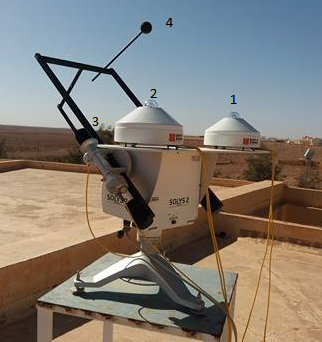Figure 1. Instrumentation station for measuring the global, direct and diffuse solar radiation: (1) pyranometer for diffuse irradiance. (2) pyranometer for global solar irradiance. (3) peryheliometer for direct irradiance component. (4) The ball used to permanently hide the pyranometer

Due to its geographical localization in the solar belt, Algeria has one of the highest solar potential in the world, namely about 5 billion (GWh/year). Sunshine duration in the Sahara and the high- lands can reach 3600 (h/year). This huge potential has pushed the authorities toward launching several projects in order to exploit this precious renewable resource.

Ghardaïa region is located in the northern part of the Algerian Sahara, about 595 km south of Mediterranean coast (Algiers), within 32°6 LN and 3°8’ LE, at an altitude of about 469 m. The area is classified as combined between arid and semi-arid zones. The annual daily average of global solar irradiance about 7 kWh/m2 at horizontal surfaces . The sunshine duration is more than 3000 h per year, which promotes the use of solar energy in various ﬁelds. The average sunshine duration between 2000 and 2009 was 3391.2 hours per year i.e. approximately 9 hours per day. Ghardaïa has a very important rate of insolation (75 % on average) and the mean annual of global solar radiation measured on horizontal plane exceeds 20 (MJ/m2). The minimum, medium and maximum average temperature levels recorded in 2015 are 6 °C, 21 °C and 40 °C.

The data are recorded every 10 min with a high precision by a radiometric station (type of pyrometer is CIMEL CES-180 with sensitivity of 12.0 ( $\mu \mathrm{V} / \mathrm{Wm}^{2}$ ), see Figure 1). Example of behavior of the two measured components (global and direct) is shown in Figures 2 and 3, respectively.

## 2.png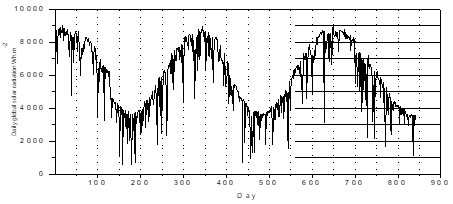Figure 2. Example of evolution of daily global radiation

## 3.png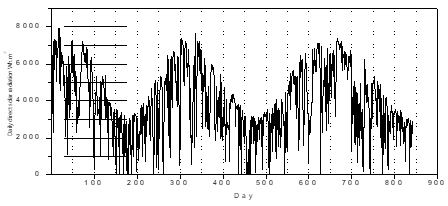Figure 3. Example of evolution of daily direct horizontal radiation

2.2 Description of longitudinal solar dryer

The studied dryer is a longitudinal dryer of indirect solar dryer type with forced convection. It is an air sensor with 8 m length, which consists of a glazing and an absorber, mounted on an insulating surface. The air passes between through the absorber and the insulating surface. The system contains three injection points through the absorbent plate by which the control of the air temperature is ensured by injecting fresh or hot air, as shown in Figure 4.

## 4.png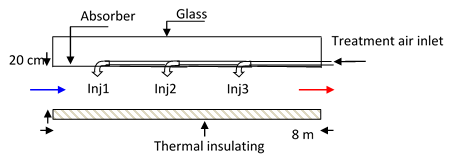Figure 4. Schematic sectional view of the longitudinal solar dryer

The solar thermal collector makes it possible to produce hot air, favoring the conversion of the global solar radiation. This energy is variable throughout the day. Figure 5 shows, the variation of the incident solar radiation on the solar air collector, at different times of the day of 08/01/2014, measured by the radiometric station with high accuracy.

The regulation rule follows the curve of the solar radiation evolutions. To be clearer at the beginning of the day when the solar irradiance is low, the regulator will act to increase the injected hot air at the inlet of thermal collector. As the solar irradiance increases, the regulator would also act to increase the amount of injected fresh air.

## 5.jpgFigure 5. Global radiation variation on a horizontal plane at Ghardaïa on Jan. 08, 2014

2.3 Mathematical formulation

The system has been simulated on the basis of simplified assumptions as following:

• The study is carried out in particular for the solar dryer channel.
• The incident solar radiation received on the absorber surface is converted to the only heat supply.
• Heat losses by convection and by conduction to the elements above the absorber will be neglected compared to the radiation losses.
• The bottom of the sensor is a perfect insulation.

The flow of a fluid, through the solar dryer cavity, is represented by a set of partial differential equations, namely the equations of the momentum and continuity. These equations obtained respectively by applying the principle of conservation of momentum and mass over an infinitesimal fluid element. Considering a laminar, stationary and two-dimensional flow of an incompressible fluid, these conservation equations are written in Cartesian coordinates as follows:

The continuity equation:

$\frac{\partial u}{\partial x}+\frac{\partial v}{\partial y}=0$  (1)

The momentum equation:

\begin{aligned} \rho\left(u \frac{\partial u}{\partial x}+v \frac{\partial u}{\partial y}\right) &=-\frac{\partial p}{\partial x}+\frac{\partial}{\partial x}\left(2 \mu \frac{\partial u}{\partial x}\right) +\frac{\partial}{\partial y}\left(\mu\left(\frac{\partial u}{\partial y}+\frac{\partial v}{\partial x}\right)\right)+\rho g_{x} \end{aligned}     (2)

\begin{aligned} \rho\left(u \frac{\partial v}{\partial x}+v \frac{\partial v}{\partial y}\right) &=-\frac{\partial p}{\partial y}+\frac{\partial}{\partial x}\left(\mu\left(\frac{\partial u}{\partial y}+\frac{\partial v}{\partial x}\right)\right) +\frac{\partial}{\partial y}\left(2 \mu \frac{\partial v}{\partial y}\right)+\rho g_{y} \end{aligned}      (3)

In the current work, volume forces will have neglected in the course of development. For non-isothermal problems, heat transfer is defined by the energy equation, which is also a result of the application of the energy conservation principle included in the fluid element.

Considering a stationary case, the fluid physical properties are independent of temperature and the energy equation will have the following form:

The energy equation:

$u \frac{\partial T}{\partial x}+v \frac{\partial T}{\partial y}=\frac{\partial}{\partial x}\left(K \frac{\partial T}{\partial x}\right)+\frac{\partial}{\partial y}\left(K \frac{\partial T}{\partial y}\right)+\Phi$ (4)

$\Phi$  Dissipation function that will be neglected.

Specific and representative boundary conditions of the physical aspect studied have been considered.

For the analysis of the hydrodynamic regime establishment:

-A non-slip condition at the walls.

-Constant speed at the inlet.

-Established speed profile at the outlet.

Whilst for the analysis of the thermal regime establishment:

-A spatiotemporal variation of the flux at the walls.

-Constant temperature at the inlet.

-Established temperature profile at the outlet.

As a generalization, the above-mentioned equations are reduced to non-dimensional form by considering the scales of length (H), velocity (Um) (average flow rate at the inlet) and temperature ΔT=(Tparence -Tinput) or ΔT=qH/k depending on the case treated.

Using the following change of variables:

$u^{*}=\frac{u}{u_{m}}, v^{*}=\frac{v}{v_{m}}, x^{*}=\frac{x}{H}, y^{*}=\frac{y}{H}$

Dimensionless pressure   $P^{*}=P /\left(\mu \frac{u_{m}}{H}\right)$

Prandt Number  $\operatorname{Pr}=\frac{c p \mu}{K}$

Reynold Number  $R e=\frac{\rho u_{m} H}{\mu}$

Peclet Number $P e=\operatorname{Pr} R e=\frac{u_{m} H}{K}$

For the case of temperature:

$\theta=\left(T-T_{e}\right) /\left(q_{r e f} \frac{R}{K}\right)$ (5)

The governing equations (1)-(4) reduce to non-dimensional form:

$\frac{\partial u^{*}}{\partial x^{*}}+\frac{\partial v^{*}}{\partial y^{*}}=0$ (6)

$\operatorname{Re}\left(u^{*} \frac{\partial u^{*}}{\partial x^{*}}+v^{*} \frac{\partial u^{*}}{\partial y^{*}}\right)=-\frac{\partial P^{*}}{\partial x^{*}}+\frac{\partial}{\partial x^{*}}\left(2 \frac{\partial u^{*}}{\partial x^{*}}\right)+\frac{\partial}{\partial y^{*}}\left(\left(\frac{\partial u^{*}}{\partial y^{*}}+\frac{\partial v^{*}}{\partial x^{*}}\right)\right)$ (7)

$\operatorname{Re}\left(u^{*} \frac{\partial v^{*}}{\partial x^{*}}+v^{*} \frac{\partial v^{*}}{\partial y^{*}}\right)=-\frac{\partial P^{*}}{\partial y^{*}}+\frac{\partial}{\partial x^{*}}\left(\left(\frac{\partial u^{*}}{\partial y^{*}}+\frac{\partial v^{*}}{\partial x^{*}}\right)\right)+\frac{\partial}{\partial y^{*}}\left(2 \frac{\partial u^{*}}{\partial y^{*}}\right)$ (8)

$P e\left(u^{*} \frac{\partial \theta^{*}}{\partial x^{*}}+v^{*} \frac{\partial \theta^{*}}{\partial y^{*}}\right)=\left\{\frac{\partial}{\partial x^{*}}\left(\frac{\partial \theta^{*}}{\partial x^{*}}\right)+\frac{\partial}{\partial y^{*}}\left(\frac{\partial \theta^{*}}{\partial y^{*}}\right)\right\}$ (9)

2.4 Numerical method

Several numerical methods offer the possibility of solving the system of partial differential equations. The finite difference method [20–24], finite volumes [25, 26], finite elements [27–29]. These methods allow the transformation of the EDP system or its integral form into a system of algebraic equations, whose resolution leads to reconstruction of the solution form.

In this study, the finite element method has been adopted and can be summarized as follows:

-Subdivision of the physical domain into a number of elements.

-Rewriting of the EDP system in an integral form by the introduction of the weighted residual method.

-Simple approximation of unknown variables in order to allow the passage from the EDP system put in integral form, to a system of algebraic equations.

-Numerical resolution of algebraic equations system.

3. Results and Discussions

It is well known that the solar drying of products (Agri-food, construction, pharmaceuticals, etc.) require a linear increase in the temperature of the drying air. This evolution will allow the gradual evaporation of the water contained in the product without burning the external surface. Through in this study, we have introduced regulatory processes that ensure, regardless of the intensity of the solar radiation, a linear evolution of the temperature between 308 K and 336 K at the base plate of the dryer a profile considered favorable to the drying process.

Depending on the variation in the intensity of the incident solar radiation on the dryer absorber, the temperature profile in question is maintained by a combination of different control modes:

• Increase or decrease the ventilation rate at the inlet of the dryer.
• Heating of ventilation air.
• Introduction of hot air by localized injections at the absorber.
• Introduction of fresh air through the same injection points.
• Variation in the flow rate of injections.

A temporal analysis of the solar radiation effect on the evolution of the drying temperature for two admissible extremes of ventilation rate (Remin=25 and Remax=85) has been carried out. Therefore, depending on the behavior of the thermal field, subdividing the time of sunshine in intervals, then adopting to each of them an appropriate regulation scenario, has been suggested.

It should be noted that for all the figures, the continuous curves represent the temperature changes with regulation and the discontinuous curves represent the temperature changes without regulation.

3.1 Beginning from 7h50' to 9h50'

This period of day is characterized by low radiation that is insufficient to rapidly initiate the drying process. To remedy this situation, an intervention is required in terms of ventilation speed. Therefore, several tests have been carried out in order to obtain the ideal margin of ventilation of the air at the entrance of the solar dryer.

• For Reynolds values less than 25, there is a rather slow flow where the conduction takes precedence over heat transfer. The thermal field reaches very high temperature values, higher than the desired values.
• For Reynolds values greater than 85, the air need to evacuated rapidly by convection, which prevents the temperature from rising.

On the basis of these two scenarios, a regulation was adopted which consists in gradually increasing the rate of ventilation from Re=25 to 85, which allowed us to have a temperature profile favorable to drying and to maintained between sunshine hours and 9:20'. Figure 6-a and figure 6-b illustrate the configuration of the thermal field with and without regulation for two distinct instants.

## 6.png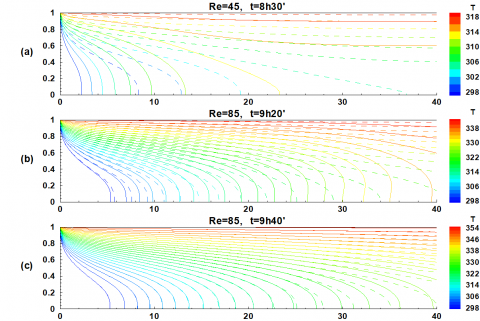Figure 6. Temperature field in the dryer (the isotherms in the channel with and without regulation)

Between 9h20' and 9h50', the Reynolds number is maintained at 85, which induces a minimal variation in the thermal field as illustrated in Figure 6-c.

Figure 7-a clearly show the effect of regulation on the longitudinal fluid temperature profiles. It can be noted that, by virtue of this, the desired values are reached within a shorter time interval.

## 7.jpg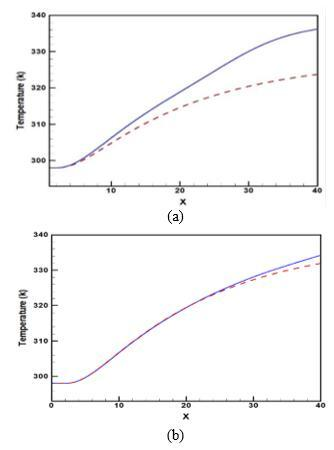Figure 7. Variation of fluid temperature ((a) for Re varied from 25 to 85 and t=8h30', (b) for Re=85 and t=9h40')

It is noted also at the varying of the Reynolds number, one reaches 336 K at 8h30' whereas this value is obtained at 9h40' without regulation and with Re=85 (Figure 7-b). A remarkable temperature difference of more than 12 °C is retained between the two cases. This testifies to the effectiveness of the regulation in terms of gain of drying time.

3.2 From 9h50' to 12h10'

Progressively, the radiation incident on the upper wall of the dryer increases causing a very significant rise in the air temperature inside the solar air heater. This can adversely affect the dried product quality. This situation is represented by Figure 8, which schematizes the structure of the thermal field for different moments. The necessity of having a temperature profile in a limited range forced us to mitigate this elevation by injecting fresh air (at a temperature of 298 K).

## 8.png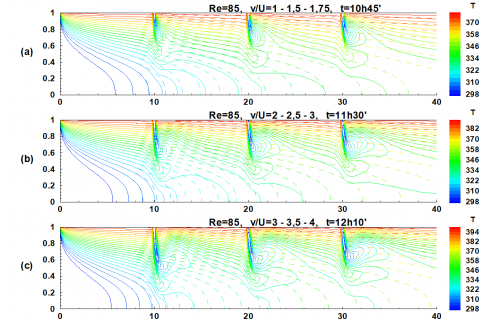Figure 8. Temperature profile in the dryer (isotherms in the channel with and without regulation)

In order to maintain the temperature in a favorable range for drying, it was determined after several simulations the number of injection points required and the associated speed values which ensure this spot. The scenario is as follows:

Table 1. Air velocity at different injection points

 v/ Ū Period Inj 1 Inj 2 Inj 3 (a) 9h55’ à 10h45’ 1 1.5 1.75 (b) 10h45’ à 11h30’ 2 2.5 3 (c) 11h30’ à 12h10’ 3 3.5 4

Without the introduction of fresh air, it is clear that the temperature reaches very high values with a threshold of 360 K as shown in Figure 8 and Figure 9 (discontinuous curve). The injection of air makes it possible to maintain the temperature gradient almost constant.

Figure 9 clearly shows the effect of this regulation through the temperature difference of 22 °C and can easily destroy the quality of the products to be dried. At the entrance, the curves representing the two evolutions of temperature, regulated and unregulated, without confused.

## 9.jpg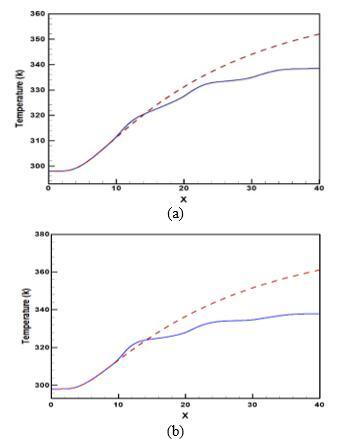Figure 9. Fluid temperature change with Re = 85.

( a) at t = 10h45' (b) at t = 11h30'

3.3 From 12:10 pm till 4:30 pm

## 10.png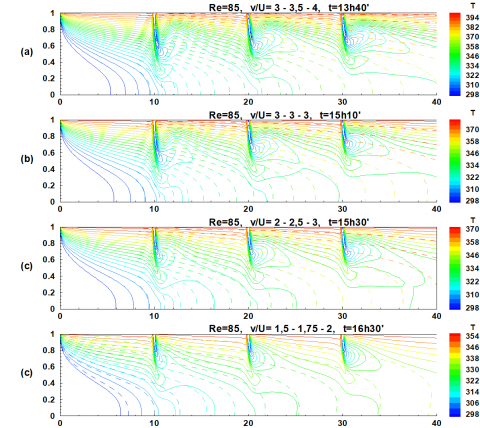Figure 10. Temperature profile in the dryer (isotherms in the channel with and without regulation)

The same work was done for this period of day. This operating period is characterized by the reduction of the radiation incident on the absorber of the solar dryer. In the absence of fresh air injection, the temperature at the sensor output decreased from 370 K to 338 K in a space of 3 hours. Despite this fact, the temperature profiles obtained are outside the desired temperature range meeting the requirements of drying.

However, the influence of the fresh air injected is remarkable on the development of the thermal field (Figure 10). This case is characterized by injection rates whose values decrease as they evolve over time. The regulation obtained is given in Table 2.

Table 2. Air velocity at different injection points

 v/Ū Period Inj 1 Inj 2 Inj 3 (a) 12h10’ à 13h40’ 3 3.5 4 (b) 13h40’ à 15h10’ 3 3 3 (c) 15h10’ à 15h40’ 2 2.5 3 (d) 15h40’ à 16h35’ 1.5 1.75 2

These velocities are important during the warm period of the day (the importance of solar radiation) and are low at the beginning and at the end of the day (low solar radiation). The results of the control illustrated in the various curves of Figure 11 show the maintenance of a temperature between 308 K and 336 K.

Temperature profiles from unregulated flow tend to the desired profile with decreased solar radiation (Figure 11-d). At this time, the injection of fresh air must cease to maintain the temperatures desired.

## 11a.jpg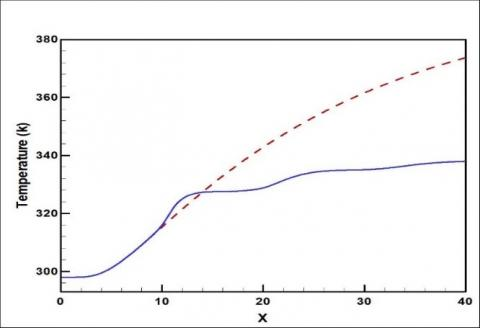(a)

## 11b.jpg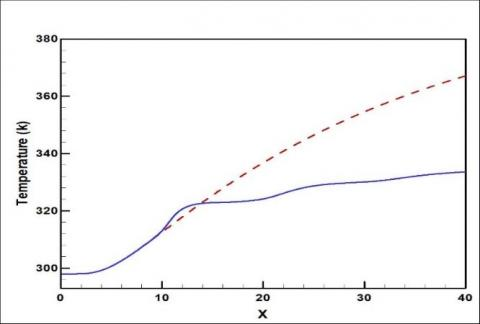(b)

## 11c.jpg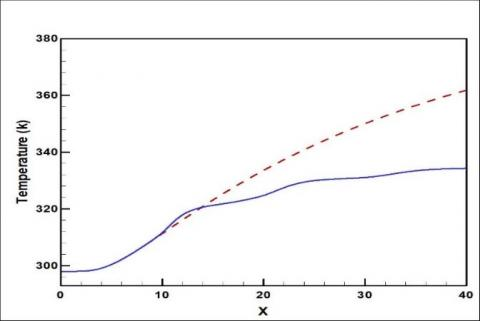(c)

## 11d.jpg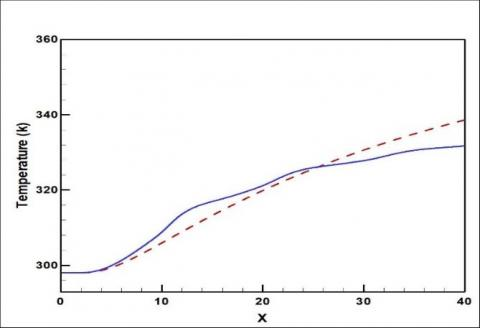(d)

Figure 11. Fluid temperature change with Re = 85; (a) at t = 13h40'; (b) at t = 15h10' (c) at t = 15h30'; (d) at t = 16h30'

3.4 From 4:30 pm until the start of the second day or the end of the process

Start from 4:30 pm, the solar radiation weakens at a point that makes it necessary to stop the injection process and reduce the ventilation rate from Re = 85 to 25. This manipulation has kept the evolution of the temperature profile within the desired range for 20 minutes (Figure 12-a).

## 12.png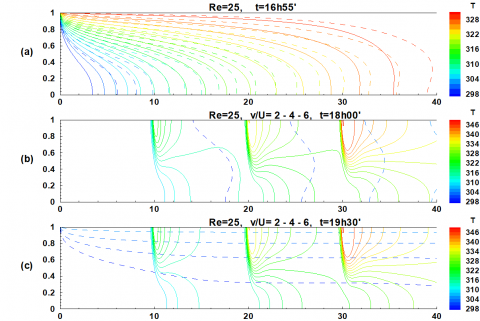Figure 12. Profile of the temperatures in the dryer (the isotherms in the channel with and without regulation)

## 13.jpg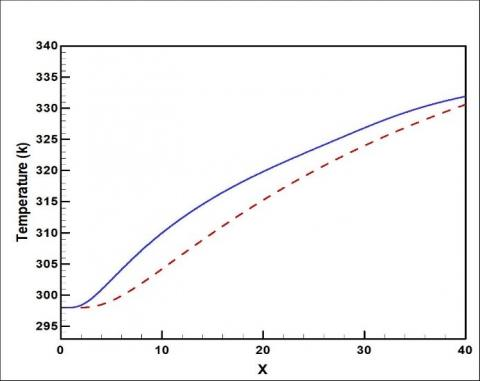Figure 13. Variation of fluid temperature with Re varies from 85 to 25; at t=16.55’

In Figure 13, it can be seen that, without regulation, temperatures decrease considerably and the obtained profile is below the desired range. This confirms the value of the regulation introduced.

Table 3. Air velocity and temperature at different injection points

 Temperature v/Ū Inj 1 328 2 Inj 2 338 4 Inj 3 348 6

## 14.jpg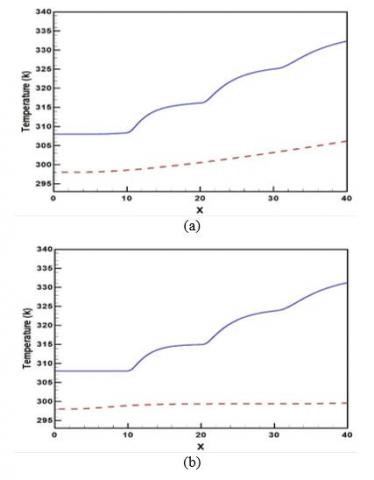Figure 14. Evolution of fluid temperatures by injecting hot air. (a) at t = 18h; (b) at t=19h30 '

After 5 pm, solar radiation is almost absent, which causes the drying process to stop, unless hot air was injected. In order to ensure the continuity of the drying process during the night, the ventilation air at the inlet of the collector was heated to 308 K and on the other hand, Injection of hot air at different temperatures and locations to reconstitute profiles favorable to the drying process. The calorific energy discharged from the dryer during the sunny period can be used in this manipulation by adding a thermal storage system to the solar dryer. The speed and temperature of the air introduced at each injection point are given in the table below.

With injections and ventilation by hot air at the inlet of the sensor, the gradient of temperature was maintained along the night period. This is illustrated in Figure 14, which also shows a very low temperature change caused by the thermal inertia of the dryer's absorbent plate and will soon disappear.

## 15.jpg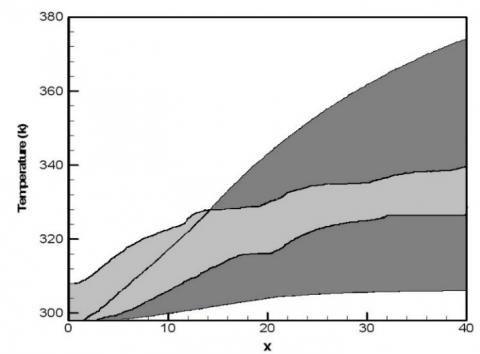Figure 15. Evolution of the air temperature along the dryer as a function of time

The curves in Figure 15 show, in a global manner, the range of temperature profiles along the dryer for the entire drying period, light gray for regulated flow and dark gray for flow without regulation. It is quite clear that the control techniques adopted have made it possible to maintain the temperature profile favorable for our drying process.

## 16.jpg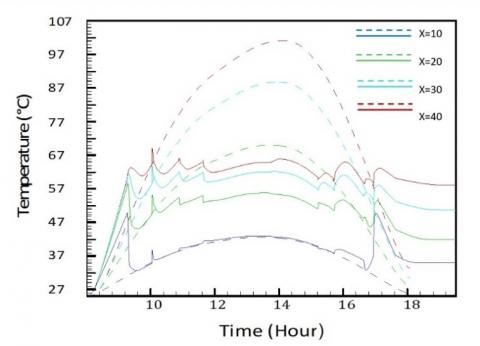Figure 16. Evolution of the temperature at positions:  x=10, x=20, x=30, x=40

The time evolution of the temperature of the fluid at points (10, 20, 30, and 40) for the entire drying period is given by figure 16. It is noted that, for a flow without regulation, the temperatures evolve in a very important range outside the desired drying range; they exceed 100 °C at the outlet of the channel. Contrary, the regulation causes the temperature to fluctuate around a distinct average value for each point. These fluctuations caused by the sudden variation of the modes of regulation attest to the maintenance of the evolution of the longitudinal temperature profile around a typical profile, which represents the ideal case for our process.

4. Conclusion

The main objective of the current investigation was to develop a mathematical model that describes the process of heat transfer through the dryer channel. Indeed, an adequate model was developed and it is described in material and method section. This model allowed control of drying temperature over the entire drying process to obtain a high quality dried product. Fundamentally, the temperature profile is maintained within the range suitable for drying regardless of the fluctuation in incoming solar radiation. Specially, at the beginning of the day, when the solar radiation intensity is low, the ventilation rate is to be maintained in a range of Reynolds number varying between Re=25 and Re=85. This enables achieving suitable drying temperature in short period of time, as the drying process progresses the Reynolds number is kept at 85. This results in a considerable saving of energy. As solar radiation intensity increases a cooling by injection of fresh air is proceeded through dedicated localized injection openings. Basically, the rate of injection is proportional to the increase of solar radiation intensity until it reaches its maximal value as the solar radiation intensity decreases the ventilation rate is accordingly decreased. At the end of the day when the solar intensity reduced which may engender a halt to drying process, a converse procedure to that at the beginning of drying is adopted i.e. the ventilation rate is varied within Reynolds number range of 85–25. In nocturnal time to avoid the interruption of the drying process, a ventilation using hot air (308 K) at the collector inlet combined with injection of hot air at different temperature is preceded. To be clearer, the injected air temperature differs from injection openings to another.

In order, to make proficient use of solar radiation resources a thermal storage system is to be appended to solar dryer to extend the drying process to low solar radiation intensity. The regulation enabled the control of the drying temperature over the entire drying time. Typically, the temperature is kept within the range suitable for dried product such that the final outcome is a high quality product.

The mathematical model proposed gives satisfaction for most numerical results obtained. This modeling can be considered as a first step towards a more rigorous modeling of the thermal transfer. This can be provided perfect stability of the temperature gradient along the drying process.

Nomenclature
 H Characteristic length of the physical domain, m K P q T t u v x y Thermal diffusivity, m2.s-1 Pressure, pa Heat flux, W.m-2 Temperature, °C Time, s Longitudinal speed, m.s-1 Cross velocity, m.s-1 Longitudinal coordinate, m Cross-sectional coordinate, m Greek symbols Ɵ Dimensionless temperature µ Dynamic viscosity, kg. m-1.s-1 ρ Density,   Kg. m-3 Subscripts e Inlet p Walls m Average
References

 Garg, H.P. (2000). Solar Energy: Fundamentals and Applications.

 Sodha, M.S., Chandra, R. (1994). Solar drying systems and their testing procedures: A review. Energy Conversion and Management, 35(3): 219-267. https://doi.org/10.1016/0196-8904(94)90004-3

 Tiwari, A. (2016). A review on solar drying of agricultural produce. Journal Food Processing & Technology, 7(9): https://doi.org/10.4172/2157-7110.1000623

 Kalogirou, S.A., Karellas, S., Braimakis, K., Stanciu, C., Badescu, V. (2016). Exergy analysis of solar thermal collectors and processes. Progress in Energy and Combustion Science, 56: 106-137. https://doi.org/10.1016/j.pecs.2016.05.002

 Pandey, K.M., Chaurasiya, R. (2017). A review on analysis and development of solar flat plate collector. Renewable and Sustainable Energy Reviews, 67: 641-650. https://doi.org/10.1016/j.rser.2016.09.078

 Shukla, A., Nkwetta, D.N., Cho, Y.J., Stevenson, V., Jones, P. (2012). A state of art review on the performance of transpired solar collector. Renewable and Sustainable Energy Reviews. 16(6): 3975-3985. https://doi.org/10.1016/j.rser.2012.02.029

 Yadav, A.S., Bhagoria, J.L. (2013). Heat transfer and fluid flow analysis of solar air heater: A review of CFD approach. Renewable and Sustainable Energy Reviews, 23: 60-79. https://doi.org/10.1016/j.rser.2013.02.035

 Benseddik, A., Azzi, A., Khanniche, R., Allaf, AK. (2018). Simulation study of solar air collector with offset strip fin absorber plate for drying agricultural products in a semi-arid climate. International Journal of Heat and Technology, 36(2): 557-568. https://doi.org/10.18280/ijht.360219

 Arunachalam, U., Edwin, M. (2017). Experimental investigations on thermal performance of solar air heater with different absorber plates. International Journal of Heat and Technology, 35(2): 393-397. https://doi.org/10.18280/ijht.350223

 Fudholi, A., Sopian, K. (2018). Review on exergy and energy analysis of solar air heater. International Journal of Power Electronics and Drive, 9(1): 420-426. https://doi.org/10.11591/ijpeds.v9.i1.pp420-426

 Saxena, A., Varun, El-Sebaii, A.A. (2015). A thermodynamic review of solar air heaters. Renewable and Sustainable Energy Reviews, 43: 863-890. https://doi.org/10.1016/j.rser.2014.11.059

 Kumar, M., Sansaniwal, S.K., Khatak, P. (2016). Progress in solar dryers for drying various commodities. Renewable and Sustainable Energy Reviews, 55: 346-360. https://doi.org/10.1016/j.rser.2015.10.158

 Saini, P., Patil, D.V., Powar, S. (2018). Review on integration of solar air heaters with thermal energy storage. Applications of Solar Energy, 163-186. https://doi.org/10.1007/978-981-10-7206-2_9

 Kabeel, A.E., Hamed, M.H., Omara, Z.M., Kandeal, A.W. (2017). Solar air heaters: design configurations, improvement methods and applications – a detailed review. Renewable and Sustainable Energy Reviews, 70: 1189-1206. https://doi.org/10.1016/j.rser.2016.12.021

 upta, A.D., Varshney, L. (2017). Performance prediction for solar air heater having rectangular sectioned tapered rib roughness using CFD. Thermal Science and Engineering Progress, 4: 122-132. https://doi.org/10.1016/j.tsep.2017.09.005

 Yadav, A.S., Samant, T.S., Varshney, L. (2015). A CFD Based Analysis of Solar Air Heater Having V-Shaped Perforated Blocks on Absorber Plate. International Research Journal of Engineering and Technology, 2(2): 822-829.

 Mathur, A., Agrawal, G.D. (2016). Thermal performance investigation and optimisation of fin type solar air heater- a CFD approach. Progress in Computational Fluid Dynamics, an International Journal, 16(1): 58. https://doi.org/10.1504/pcfd.2016.074220

 Gawande, V.B., Dhoble, A.S., Zodpe, D.B., Chamoli, S. (2016). Experimental and CFD investigation of convection heat transfer in solar air heater with reverse L-shaped ribs. Sol. Energy, 131: 275-295. https://doi.org/10.1016/j.solener.2016.02.040

 Lalmi, D., Bezari, S., Bensaha, H., Guermouai, M., Rabehi, A., Abdelouahab, B., Hadef, R. (2018). Analysis of thermal performance of an agricultural greenhouse heated by a storage system. Model. Meas. Control B, 87(1): 15-20. https://doi.org/10.18280/mmc_b.870103

 Forsythe, G., Wasow, W. (1960). Finite-difference Methods For Partial Differential Equations. Applied Mathematical Series. Wiley, New York.

 Özışık, M.N. (1994). Finite Difference Methods In Heat Transfer. CRC Press. https://doi.org/10.1201/9781315168784

 Richtmyer, R.D., Morton, K.W. (1994). Difference methods for initial-value problems. Physics Today, 12(4): 50. https://doi.org/10.1063/1.3060778

 Smith, G.D., Gordon, D. (1985). Numerical Solution Of Partial Differential Equations : Finite Difference Methods. Clarendon Press.

 Strikwerda, J.C. (1983). Finite Difference Methods for the Incompressible Navier-Stokes Equations – A Survey. MR Tech. Summ. Rep. #2584, Math. Res. Cent. Univ. Wisconsin.

 Patankar, S. (1980). Numerical Heat Transfer and Fluid Flow. Hemisphere Pub. Corp.

 Versteeg, H.K., Malalasekera, W. (2007). An Introduction to Computational Fluid Dynamics: The Finite Volume Method. Pearson Education Ltd.

 Baker, A.J. (1983). Finite Element Computational Fluid Mechanics. Hemisphere Pub. Corp.

 Chung, T.J. (1978). Finite Element Analysis in Fluid Dynamics. McGraw-Hill International Book Co.

 Zienkiewicz, O.C. (1977). The Finite Element Method. London; New York: McGraw-Hill.